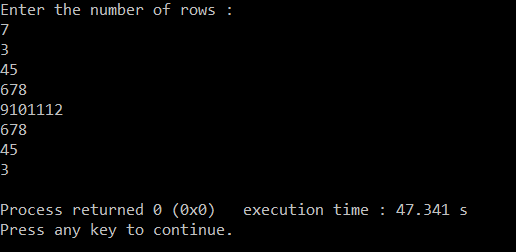# Print Right Diamond Number Pattern Type5

3

45

678

9101112

678

45

3

## PREREQUISITE:

Basic knowledge of C language and use of loops.

## ALGORITHM:

1. Take the number of rows as input from the user and store it in any variable.(‘r‘ in this case).
2. Run a loop ‘r’ number of times to iterate through each of the rows. From i=0 to i<r. The loop should be structured as for( i=0 ; i<r : i++).
3. Use an if condition to to print the top half of the pyramid. if (i<=r/2). Then intialise count1=count+1 and Then run a loop from j=0 to j<=i. The loop should be structured as for(j=0 ; j<=i ; j++)
4. Inside this loop increment count and then print it.
5. Outside this loop print a newline.
6. Else count=count1-(r-i); and count1=count(to print the values accordingly).
7. Run a different loop from j=i to j<r. The loop should be structured as for( j=i ; j<r ; j++).
8. Inside this loop print count and then increment it.
9. Inside the main loop print a newline to move to the next line after each row is printed.

## CODE IN C:

```#include<stdio.h>
int main()
{
int i,j,r,count,count1;                 //declaring integer variables i,j for loops , r for number of rows and count and count1 for increment in value
count=2; //initialising count
printf("Enter the number of rows :\n"); //Asking user for input
scanf("%d",&r);                         //taking number of rows and saving it in variable r
for(i=0;i<r;i++)                        // loop for number of rows
{
if(i<=r/2)                           //if statement to print top half
{
count1=count+1;                   //giving the changed value to count1
for(j=0;j<=i;j++)                 // loop for digits per each row
{
count++;                   //incrementing count
printf("%d",count);        //printing digits
}
printf("\n");                    // printing newline after each row

}
else                                 //else statement for bottom half
{
count=count1-(r-i);               //reevaluating count
count1=count;                     //giving value of count to count1
for(j=i;j<r;j++)                  //loop for bottom half
{
printf("%d",count);         //printing count
count++;                    //incrementing count
}
printf("\n");                        //printing newline
}

}

}```

## DISPLAYING OUTPUT: